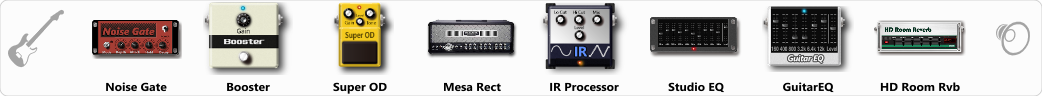# IM BROKEN

Discussion in 'ToneLib-GFX presets' started by Lester Falcon, Sep 11, 2019.

1. IM BROKEN

Preset name: Im broken

Effects chain:Effect: "Noise Gate" (Dynamics / Filter), active - "yes"
"Detect" = Gtr In
"Mode" = Manual
"Depth" = 50
"Threshold" = 50
"Attack" = 0
"Hold" = 5
"Decay" = 250

Effect: "Booster" (Dynamics / Filter), active - "yes"
"Gain" = 25

Effect: "Super OD" (Overdrive / Distortion), active - "yes"
"Drive" = 8
"Tone" = 80
"Level" = 95

Effect: "Mesa Rect" (Amp simulators), active - "yes"
"Gain" = 30
"Bass" = 60
"Middle" = 46
"Treble" = 60
"Presence" = 50
"Master" = 50
"Output" = 50
"Level (dB)" = 5

Effect: "IR Processor" (Dynamics / Filter), active - "yes"
"IR" = PanteraVulgarDisplayOfPower
"Low Cut (Hz)" = 0
"Hi Cut (kHz)" = 20.0
"Mix" = 100
"Level (dB)" = 0

Effect: "Studio EQ" (Dynamics / Filter), active - "yes"
"31 Hz" = -3
"62 Hz" = -2
"125 Hz" = 5
"250 Hz" = 3
"500 Hz" = 0
"1 kHz" = 2
"2 kHz" = 0
"4 kHz" = 2
"8 kHz" = -1
"16 kHz" = 0
"above 16 kHz" = 0
"Level (dB)" = 0

Effect: "GuitarEQ" (Dynamics / Filter), active - "yes"
"160 Hz" = 0
"400 Hz" = 4
"800 Hz" = 4
"1.6 kHz" = 4
"3.2 kHz" = -1
"6.4 kHz" = 5
"12 kHz" = -2
"Level (dB)" = 0

Effect: "HD Room Rvb" (Reverb), active - "yes"
"Time" = 6.6
"PreLPF" = 77
"PreDelay" = 60
"HiDamp" = 65
"LoGain" = -3.5
"Mix" = 19

Note: You will need to download and install the ToneLib-GFX software to use the preset.

#### Attached Files:

• ###### IM_BROKEN.tlgfx
File size:
3.5 KB
Views:
4,356
vasia, TKEVI, Toprak and 8 others like this.